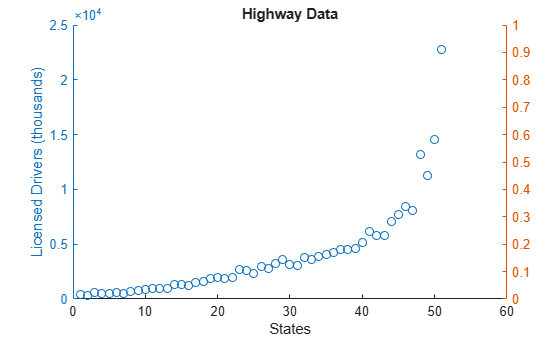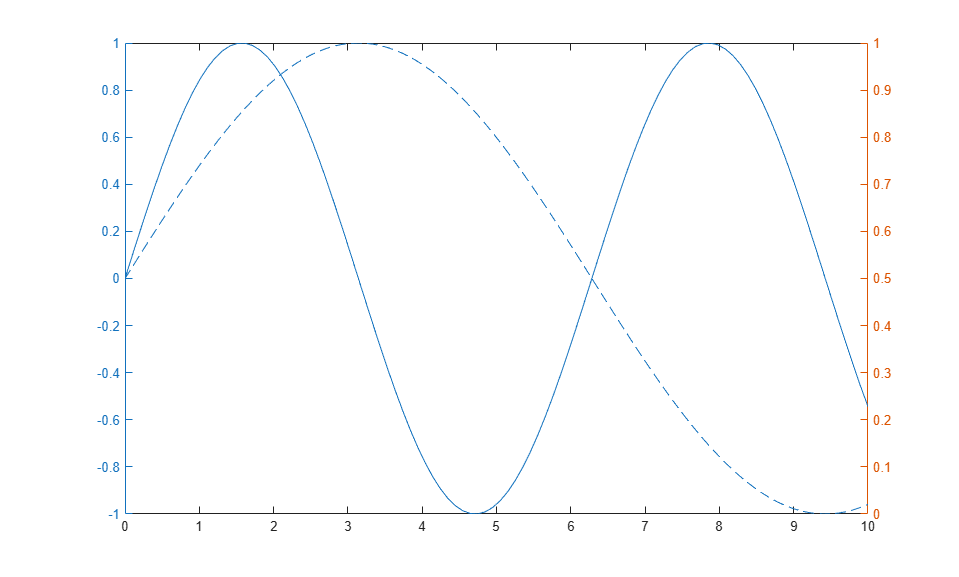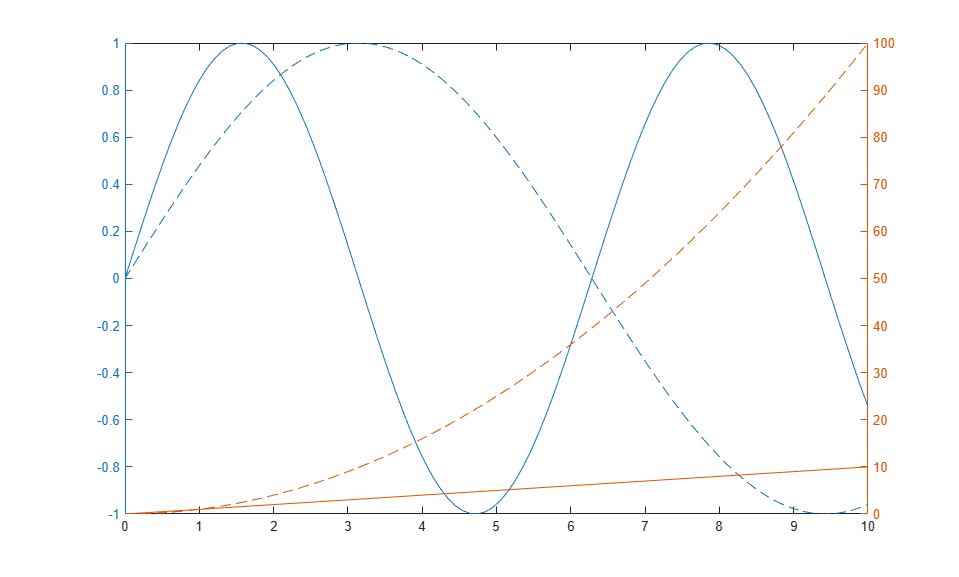# yyaxis

## 语法

``yyaxis left``
``yyaxis right``
``yyaxis(ax,___)``

## 说明

``yyaxis left` 激活当前坐标区中与左侧 y 轴关联的一侧。后续图形命令的目标为左侧。如果当前坐标区中没有两个 y 轴，此命令将添加第二个 y 轴。如果没有坐标区，此命令将首先创建坐标区。`
``yyaxis right` 激活当前坐标区中与右侧 y 轴关联的一侧。后续图形命令的目标为右侧。`

``yyaxis(ax,___)` 指定 `ax` 坐标区（而不是当前坐标区）的活动侧。如果坐标区中没有两个 y 轴，此命令将添加第二个 y 轴。指定坐标区作为第一个输入参数。使用单引号将 `'left'` 和 `'right'` 引起来。`

## 示例

```x = linspace(0,10); y = sin(3*x); yyaxis left plot(x,y) z = sin(3*x).*exp(0.5*x); yyaxis right plot(x,z) ylim([-150 150])``````load('accidents.mat','hwydata') ind = 1:51; drivers = hwydata(:,5); yyaxis left scatter(ind,drivers) title('Highway Data') xlabel('States') ylabel('Licensed Drivers (thousands)')``````pop = hwydata(:,7); yyaxis right scatter(ind,pop) ylabel('Vehicle Miles Traveled (millions)')``````x = linspace(0,10); yl1 = sin(x); yl2 = sin(x/2); yyaxis left plot(x,yl1) hold on plot(x,yl2) ``````yr1 = x; yr2 = x.^2; yyaxis right plot(x,yr1) plot(x,yr2) hold off ``````yyaxis left cla ``````colororder({'b','m'}) yyaxis left y = [1 2; 3 4]; plot(y) yyaxis right z = [4 3; 2 1]; plot(z) legend``````yyaxis left bar(magic(3)); colororder('default')```

```yyaxis right scatter([1 2 3],[2 5 2],'filled') hold on scatter([1 2 3],[3 4 1],'filled') scatter([1 2 3],[4 2 4],'filled') hold off colororder({'r','b','c'}) legend``````x = linspace(1,10); tiledlayout(2,1) % Top plot ax1 = nexttile; yyaxis(ax1,'left') plot(ax1,x,sin(x)) yyaxis(ax1,'right') plot(ax1,x,exp(x)) % Bottom plot ax2 = nexttile; plot(ax2,1:10)```## 局限性

• 使用两个 y 轴时，您不能：

• 旋转坐标区（仅适用于二维视图）。

• 固定注释。

• 使用 `copyobj` 复制坐标区对象。

## 提示

• 要确定坐标区的哪一侧处于活动状态，请查询 `Axes` 对象的 `YAxisLocation` 属性。当左侧处于活动状态时，此属性设置为 `'left'`；当右侧处于活动状态时，此属性设置为 `'right'`。有两个 y 轴的 `Axes` 对象的 `YAxisLocation` 属性为只读属性。

• 要清除活动侧，请使用 `cla`。要清除坐标区的两侧并删除右侧 y 轴，请使用 `cla reset`。也可以通过将 `Axes` 对象的 `NextPlot` 属性设置为 `'replaceall'`，等到执行下一个绘图命令时再清除坐标轴的两侧并删除右侧 y 轴。

• `Axes` 对象的 `Children` 属性只包含活动侧的子级。要访问两侧的所有子级，请使用 `allchild` 函数。

## 算法

### 轴属性

y 轴有关的坐标区属性有两个值。但是，MATLAB® 只允许访问活动侧的值。例如，如果左侧处于活动状态，则 `Axes` 对象的 `YLim` 属性包含左侧 y 轴的范围。不过，如果右侧处于活动状态，则 `YLim` 属性包含右侧 y 轴的范围。